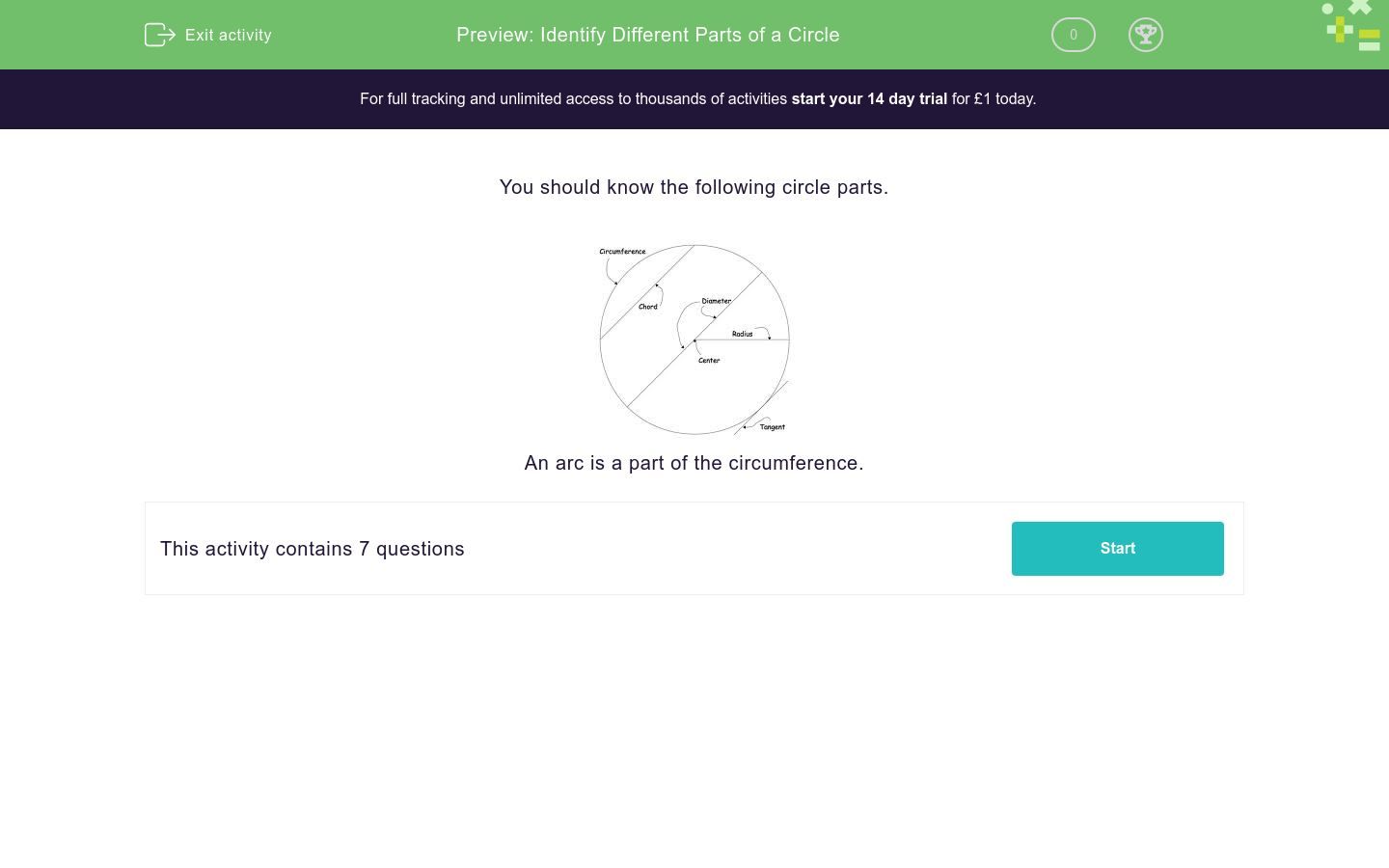# Parts of a Circle

In this worksheet, students learn the names of the parts of a circle.Key stage:  KS 4

Curriculum topic:  Geometry and Measures

Curriculum subtopic:  Identify and Apply Circle Definitions and Properties

Difficulty level:### QUESTION 1 of 10

You should know the following circle parts.An arc is a part of the circumference.

Complete the following sentence about the parts of a circle.The line XY is a _____________ of the circle.
Arc

Chord

Tangent

Circumference

Complete the following sentence about the parts of a circle.The distance all the way round the outside of a circle is called the __________________.
Arc

Chord

Tangent

Circumference

Complete the following sentence about the parts of a circle.The line AB is a _____________ of the circle.
Arc

Chord

Tangent

Circumference

Complete the following sentence about the parts of a circle.The curved part ST of the circle is called an ________.
Arc

Chord

Tangent

Circumference

Complete the following sentence about the parts of a circle.The point O is the ___________ of the circle.
Centre

Diameter

Arc

Radius

Complete the following sentence about the parts of a circle.The line MN is a _____________ of the circle.
Centre

Diameter

Diagonal

Radius

Complete the following sentence about the parts of a circle.The line OP is a _____________ of the circle.
Centre

Diameter

Arc

Radius

• Question 1

Complete the following sentence about the parts of a circle.The line XY is a _____________ of the circle.
CORRECT ANSWER
Tangent
• Question 2

Complete the following sentence about the parts of a circle.The distance all the way round the outside of a circle is called the __________________.
CORRECT ANSWER
Circumference
• Question 3

Complete the following sentence about the parts of a circle.The line AB is a _____________ of the circle.
CORRECT ANSWER
Chord
• Question 4

Complete the following sentence about the parts of a circle.The curved part ST of the circle is called an ________.
CORRECT ANSWER
Arc
• Question 5

Complete the following sentence about the parts of a circle.The point O is the ___________ of the circle.
CORRECT ANSWER
Centre
• Question 6

Complete the following sentence about the parts of a circle.The line MN is a _____________ of the circle.
CORRECT ANSWER
Diameter
• Question 7

Complete the following sentence about the parts of a circle.The line OP is a _____________ of the circle.
CORRECT ANSWER
Radius
---- OR ----

Sign up for a £1 trial so you can track and measure your child's progress on this activity.

### What is EdPlace?

We're your National Curriculum aligned online education content provider helping each child succeed in English, maths and science from year 1 to GCSE. With an EdPlace account you’ll be able to track and measure progress, helping each child achieve their best. We build confidence and attainment by personalising each child’s learning at a level that suits them.

Start your £1 trial